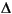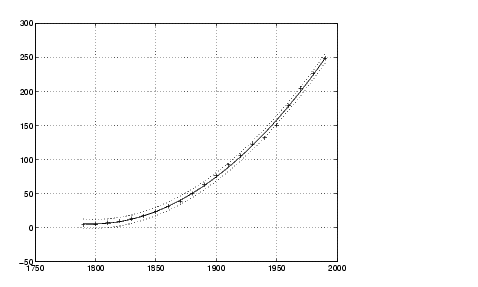MathematicsError Bounds

Error bounds are useful for determining if your data is reasonably modeled by the fit. You can obtain the error bounds by passing an optional second output parameter from `polyfit` as an input parameter to `polyval`.

This example uses the `census` demo data and normalizes the data by centering it at zero mean and scaling it to unit standard deviation. The example then uses `polyfit` and `polyval` to produce error bounds for a second-order polynomial model. Year values are normalized. This code uses an interval of ±2, corresponding to a 95% confidence interval.

• ```load census
sdate = (cdate - mean(cdate))./std(cdate)

[p2,S2] = polyfit(sdate,pop,2);
[pop2,del2] = polyval(p2,sdate,S2);
```plot(cdate,pop,'+',cdate,pop2,'g-',cdate,pop2+2*del2,'r:',...
```   cdate,pop2-2*del2,'r:'), grid on```Exponential Fit The Basic Fitting Interface© 1994-2005 The MathWorks, Inc.